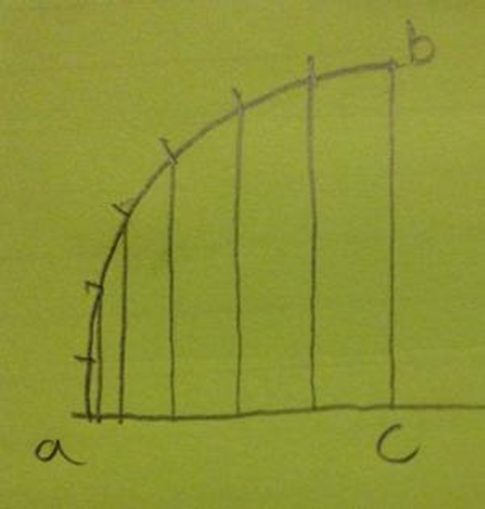# Animation

Ages ago I figured out what to do if you wanted to cut two video scenes together and have them moving at the same pace.
I thought you could do some bit of film where the movement tied it all together.
This is really for flat things I don’t know anything about matrixes. It’s easiest with those constant zooms where the area changes by the same proportion.
if x is a size and p is a proportion (like 0.02467)
frame 1: x
frame 2: x-x*p
frame 3:(x-x*p)-((x-x*p)*p)
frame 4: (x-x*p)-((x-x*p)*p)-((x-x*p)-((x-x*p)*p))*p
and so on
I missed out some of the brackets so it’s more legible but you see what I mean.
that works out to be; for the smaller frame size

small = sqrt((x^2)*((1-p)^frames))

and from that you can get the proportion, the frames and the sizes and make a crude little program to show the one that’s missing if you know three of them. ( frames, proportion, big, small )

#!/usr/bin/perl
### USAGE change the numbers, make = 0 the one you want to know ###
use strict;
use feature “say”;
use Math::Trig;

my \$small =0;
my \$big = 400;
my \$frames = 20;
my \$prop = 0.0234;

if(\$prop==0){
my \$see_prop = 1-((\$small**2)/(\$big**2))**(1/\$frames);
say ” proportion = \$see_prop”;
}
if(\$small==0){
my \$see_small = int(sqrt((\$big**2)*((1-\$prop)**\$frames)));
say ” small = \$see_small”;
}
if(\$big==0){
my \$see_big = int(sqrt((\$small**2)/((1-\$prop)**\$frames)));
say ” big = \$see_big”;
}
if(\$frames==0){
my \$see_frames = int((log(\$small**2/\$big**2))/(log(1-\$prop)));
say ” frames = \$see_frames “;
}

you can see what size the image will be at any frame in a movement over frames or where you should start to end on a size or how many frames you need on a different artwork to arrive at a size and stuff like that. And to make a zoom over a series of drawings you crop each one as so and blow them up all to the same size.

Rather than starting off or ending at a constant pace it easier on the eye if things start slowly or end slowly, that’s “fairings”
You can work out fairings if you look at it like this.if ac is the frames you want as fairings imagine an arc of a circle with radius ac
you see if you drop lines down the gaps on the baseline get progressively bigger.
What we are saying is if you have something that is moving at a constant pace and you have another thing that you want to be moving at that same pace at some point c but start off slower then that thing is going to have to start earlier and take more frames to catch up. that will be (pi*ac)/2 frames
The problem that we have is that we are dealing with frames which are whole numbers and (pi*ac)/2 is not a whole number but never mind.
Find the new proportion using size at end of fairings as the small size.
my \$see_prop = 1-((\$small**2)/(\$big**2))**(1/((pi*ac)/2));
You can loop through the fairings to get a decimal that starts small and gets bigger until it is 1 using cosine of the angle.
“faired_frame_increments=1-(cos(frame/total_faired_frames)*90)”
then multiply the new proportion by that for each frame.
Then the thing starting slowly will, after more frames, be in the same place as the thing starting later at a constant pace.
because (pi*ac)/2 isn’t a whole number I bodge it by averaging the next to last of the fairings and the size after the fairings. So if you want to match exactly you’d match to the one after the fairings end ( or start for end fairings).
Maybe there is a better way to do it I don’t know.

I struggled with tying any left right, up down movement with the zoom movement.
Dividing the track distance by the total zoom movement is a fraction, if you multiply that by the zoom movement for each frame, when it is the total zoom movement the result will be 1 * the track distance, which is what you want.